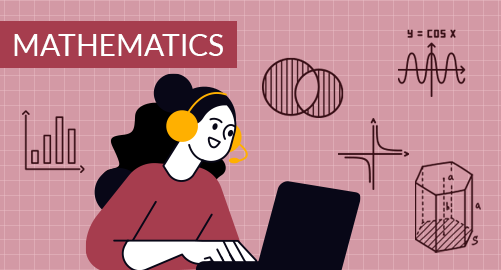#### through a demo today# Year 7 Mathematics

Mathematics
Year 7

The Year 7 Mathematics curriculum equips students with a variety of skills that are essential for mathematical development and applying mathematical concepts to real-life situations. It covers topics from numbers, fractions, decimals, indices, money and financial mathematics, time and measurements, geometry, statistics, probability, and algebra.

## What you'll learn

• 1

Number system

• 2

Factors and Multiples

• 3

Fractions, decimals, percentages

• 4

Money and financial mathematics

• 5

Geometry

• 6

Measurements

• 7

Statistics and Probability

• 8

Algebra

• 9

Linear equations

## Skills you'll learnNumeracy skills - Place values and operations with numbers, estimate, and round off sum, difference, product, and quotient, evaluation of multiple operations, and solving with brackets.Number properties - Factors and multiples of numbers, highest common factor and lowest common multiple of numbers, prime numbers, and composite numbers, prime factorisation, powers, and roots.Arithmetic operations - Basic arithmetic operations on fractions, decimals, equivalent and simplified fractions, the connection between fractions, decimals, and percentages, conversion of fractions, and decimals into percentages, and vice versa., using fractions and percentages to compare two quantities.Money and financial - Change in percentage, percentage of a sum, understanding basic financial concepts, and calculating tax and discount.Elements of geometry - Lines and angles, measuring and constructing angles, properties of 2d & 3d shapes, polygons, angle sum property, symmetry, reflection, and translation, and nets.Measurement skills - Measuring length, area, mass, and temperature. Conversion of units and measurement. Parts of circles and measuring of circles.Data analysis and interpretation - Understanding the data and drawing the conclusions, graphs, and charts. Calculate and measure central tendency (mean, median, and mode). Understanding the chance and probability, the difference between theoretical probability and experimental probability.Problem-Solving Skills - Breaking down complex problems, solving real-life problems, and making logical decisions.Algebraic thinking - Understanding algebraic expressions and equations. Solving equations and inequations, understanding planes, and drawing graphs.Analytical thinking - Solving equations, usage of formulas, understanding equality and inequality of relations, the graph of slope, variations.